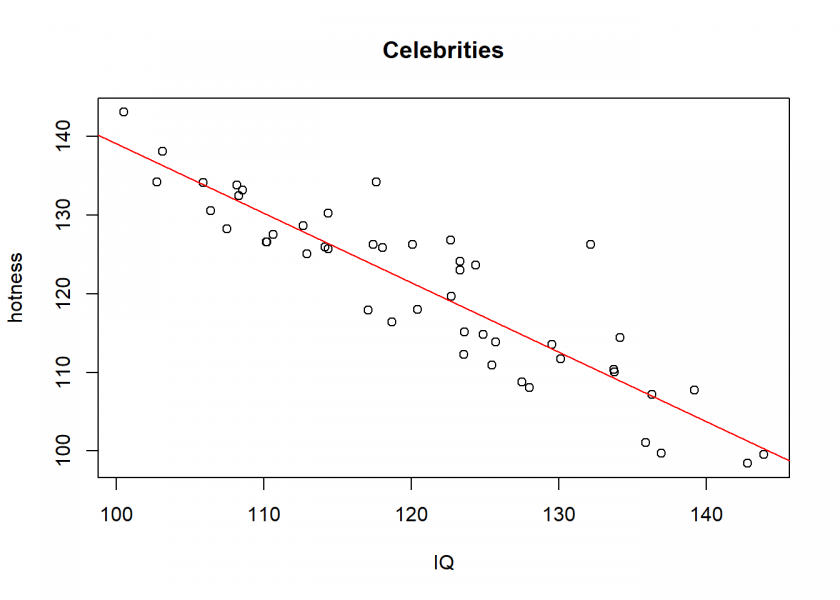Want to share your content on R-bloggers? click here if you have a blog, or here if you don't.Correlation and its associated challenges don’t lose their fascination: most people know that correlation doesn’t imply causation, not many people know that the opposite is also true (see: Causation doesn’t imply Correlation either) and some know that correlation can just be random (so-called spurious correlation).

If you want to learn about a paradoxical effect nearly nobody is aware of, where correlation between two uncorrelated random variables is introduced just by sampling, read on!

Let us just get into an example (inspired by When Correlation Is Not Causation, But Something Much More Screwy): for all intents and purposes let us assume that appearance and IQ are normally distributed and are uncorrelated:

set.seed(1147)
hotness <- rnorm(1000, 100, 15)
IQ <- rnorm(1000, 100, 15)
pop <- data.frame(hotness, IQ)
plot(hotness ~ IQ, main = "The general population")Now, we can ask ourselves: why does somebody become famous? One plausible assumption (besides luck, see also: The Rich didn’t earn their Wealth, they just got Lucky) would be that this person has some combination of attributes. To stick with our example, let us assume some combination of hotness and intelligence and let us sample some “celebrities” on the basis of this combination:

pop$comb <- pop$hotness + pop$IQ # some combination of hotness and IQ celebs <- pop[pop$comb > 235, ] # sample celebs on the basis of this combination
plot(celebs$hotness ~ celebs$IQ, xlab = "IQ", ylab = "hotness",  main = "Celebrities")
abline(lm(celebs$hotness ~ celebs$IQ), col = "red")Wow, a clear negative relationship between hotness and IQ! Even a highly significant one (to understand significance, see also: From Coin Tosses to p-Hacking: Make Statistics Significant Again!):

cor.test(celebs$hotness, celebs$IQ) # highly significant
##
##  Pearson's product-moment correlation
##
## data:  celebs$hotness and celebs$IQ
## t = -14.161, df = 46, p-value < 2.2e-16
## alternative hypothesis: true correlation is not equal to 0
## 95 percent confidence interval:
##  -0.9440972 -0.8306163
## sample estimates:
##       cor
## -0.901897


How can this be? Well, the basis (the combination of hotness and IQ) on which we sample from our (uncorrelated) population is what is called a collider (variable) in statistics. Whereas a confounder (variable) influences (at least) two variables (A ← C → B), a collider is the opposite: it is influenced by (at least) two variables (A → C ← B).

In our simple case, it is the sum of our two independent variables. The result is a spurious correlation introduced by a special form of selection bias, namely endogenous selection bias. The same effect also goes under the name Berkson’s paradox, Berkson’s fallacy, selection-distortion effect, conditioning on a collider (variable), collider stratification bias, or just collider bias.

To understand this effect intuitively we are going to combine the two plots from above:

plot(hotness ~ IQ, main = "The general population & Celebrities")
points(celebs$hotness ~ celebs$IQ, col = "red")
abline(a = 235, b = -1, col = "blue")In reality, things are often not so simple. When you google the above search terms you will find all kinds of examples, e.g. the so-called obesity paradox (an apparent preventive effect of obesity on mortality in individuals with cardiovascular disease (CVD)), a supposed health-protective effect of neuroticism or biased deep learning predictions of lung cancer.

As a takeaway: if a statistical result implies a relationship that seems too strange to be true, it possibly is! To check whether collider bias might be present check if sampling was being conducted on the basis of a variable that is influenced by the variables that seem to be correlated! Otherwise, you might not only falsely conclude that beautiful people are generally stupid and intelligent people ugly…